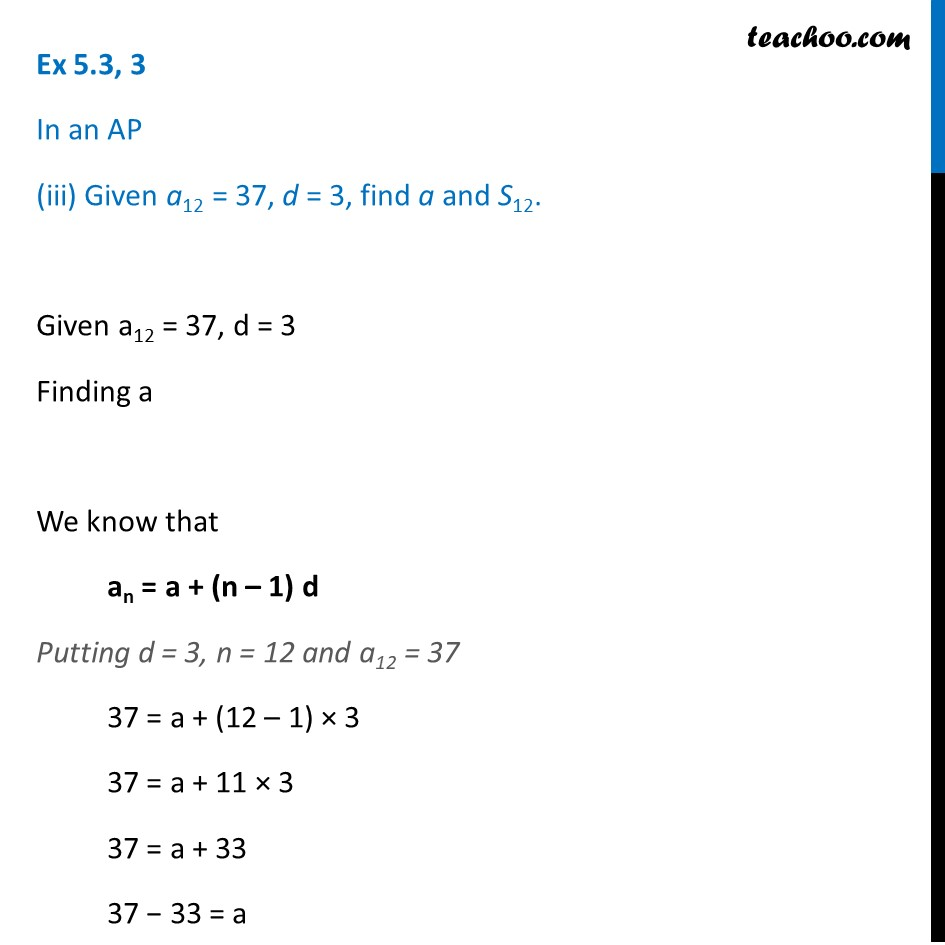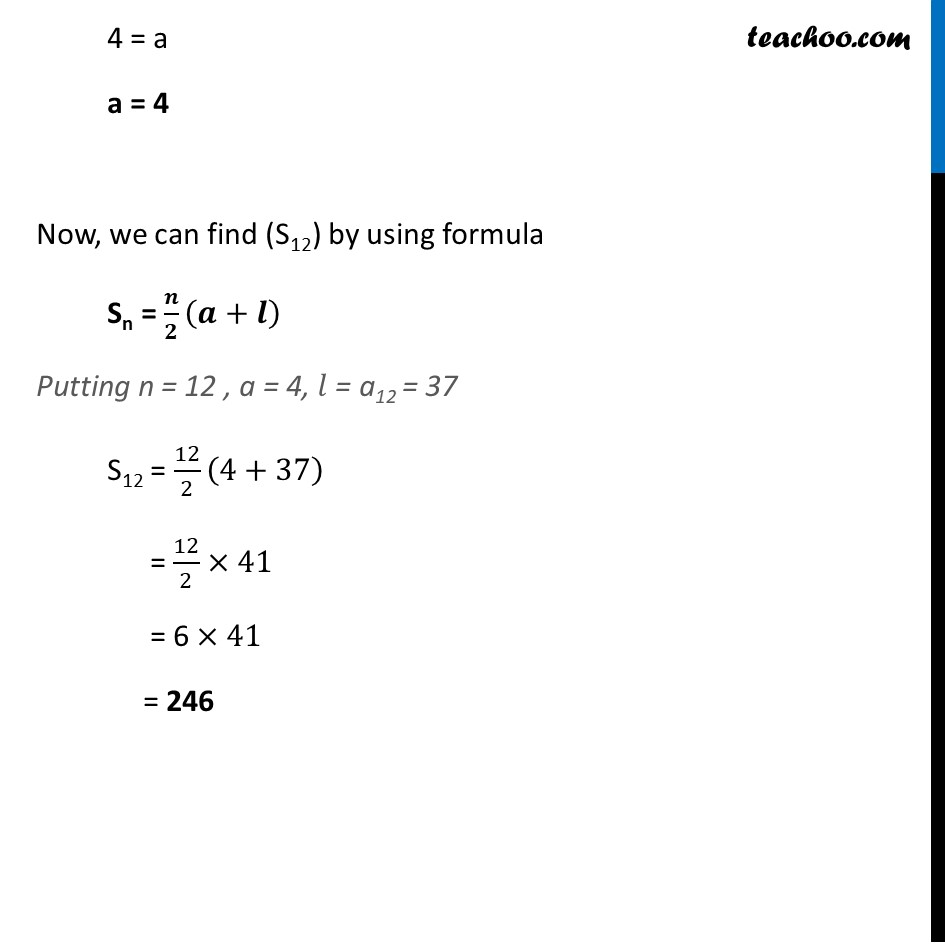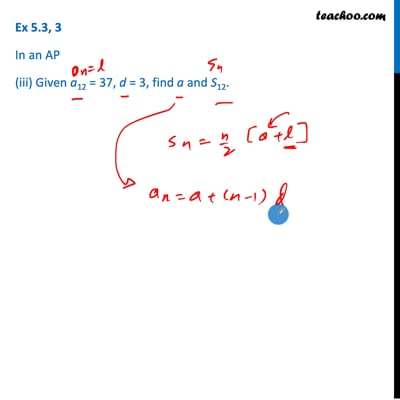Ex 5.3

Chapter 5 Class 10 Arithmetic Progressions (Term 2)
Serial order wiseThis video is only available for Teachoo black users

### Transcript

Ex 5.3, 3 In an AP (iii) Given a12 = 37, d = 3, find a and S12. Given a12 = 37, d = 3 Finding a We know that an = a + (n – 1) d Putting d = 3, n = 12 and a12 = 37 37 = a + (12 – 1) × 3 37 = a + 11 × 3 37 = a + 33 37 − 33 = a 4 = a a = 4 Now, we can find (S12) by using formula Sn = 𝒏/𝟐 (𝒂+𝒍) Putting n = 12 , a = 4, 𝑙 = a12 = 37 S12 = 12/2 (4+37) = 12/2×41 = 6 ×41 = 246